## 83. Use of General Datatypes in CommunicationUp: Derived Datatypes Next: Correct Use of Addresses Previous: Duplicating a Datatype

Handles to derived datatypes can be passed to a communication call wherever a datatype argument is required. A call of the form MPI_SEND(buf, count, datatype, ...), where, is interpreted as if the call was passed a new datatype which is the concatenation of count copies of datatype. Thus, MPI_SEND(buf, count, datatype, dest, tag, comm) is equivalent to,

```MPI_TYPE_CONTIGUOUS(count, datatype, newtype)
MPI_TYPE_COMMIT(newtype)
MPI_SEND(buf, 1, newtype, dest, tag, comm)
MPI_TYPE_FREE(newtype).
```
Similar statements apply to all other communication functions that have a count and datatype argument.

Suppose that a send operation MPI_SEND(buf, count, datatype, dest, tag, comm) is executed, where datatype has type map,

{(type0, disp0),...,(typen-1, dispn-1)},

and extent extent. (Explicit lower bound and upper bound markers are not listed in the type map, but they affect the value of extent.) The send operation sendsentries, where entry i · n + j is at location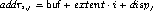and has type typej, for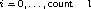and j = 0 ,..., n-1. These entries need not be contiguous, nor distinct; their order can be arbitrary.

The variable stored at address addri,j in the calling program should be of a type that matches typej, where type matching is defined as in Section Type Matching Rules . The message sent containsentries, where entry i · n +j has type typej.

Similarly, suppose that a receive operation MPI_RECV(buf, count, datatype, source, tag, comm, status) is executed, where datatype has type map, {(type0, disp0) ,...,(typen-1, dispn-1) }, with extent extent. (Again, explicit lower bound and upper bound markers are not listed in the type map, but they affect the value of extent.) This receive operation receivesentries, where entry i · n + j is at location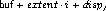and has type typej. If the incoming message consists of k elements, then we must have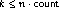; the i · n + j-th element of the message should have a type that matches typej.

Type matching is defined according to the type signature of the corresponding datatypes, that is, the sequence of basic type components. Type matching does not depend on some aspects of the datatype definition, such as the displacements (layout in memory) or the intermediate types used.

Example This example shows that type matching is defined in terms of the basic types that a derived type consists of.

```...
CALL MPI_TYPE_CONTIGUOUS(2, MPI_REAL, type2, ...)
CALL MPI_TYPE_CONTIGUOUS(4, MPI_REAL, type4, ...)
CALL MPI_TYPE_CONTIGUOUS(2, type2, type22, ...)
...
CALL MPI_SEND(a, 4, MPI_REAL, ...)
CALL MPI_SEND(a, 2, type2, ...)
CALL MPI_SEND(a, 1, type22, ...)
CALL MPI_SEND(a, 1, type4, ...)
...
CALL MPI_RECV(a, 4, MPI_REAL, ...)
CALL MPI_RECV(a, 2, type2, ...)
CALL MPI_RECV(a, 1, type22, ...)
CALL MPI_RECV(a, 1, type4, ...)
```
Each of the sends matches any of the receives.

A datatype may specify overlapping entries. The use of such a datatype in a receive operation is erroneous. (This is erroneous even if the actual message received is short enough not to write any entry more than once.)

Suppose that MPI_RECV(buf, count, datatype, dest, tag, comm, status) is executed, where datatype has type map, {(type0, disp0) ,...,(typen-1, dispn-1) }. The received message need not fill all the receive buffer, nor does it need to fill a number of locations which is a multiple of n. Any number, k, of basic elements can be received, where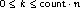. The number of basic elements received can be retrieved from status using the query functions MPI_GET_ELEMENTS or MPI_GET_ELEMENTS_X.

 MPI_GET_ELEMENTS(status, datatype, count) IN status return status of receive operation (Status) IN datatype datatype used by receive operation (handle) OUT count number of received basic elements (integer)

int MPI_Get_elements(const MPI_Status *status, MPI_Datatype datatype, int *count)

MPI_Get_elements(status, datatype, count, ierror)
TYPE(MPI_Status), INTENT(IN) :: status
TYPE(MPI_Datatype), INTENT(IN) :: datatype
INTEGER, INTENT(OUT) :: count
INTEGER, OPTIONAL, INTENT(OUT) :: ierror
MPI_GET_ELEMENTS(STATUS, DATATYPE, COUNT, IERROR)
INTEGER STATUS(MPI_STATUS_SIZE), DATATYPE, COUNT, IERROR

 MPI_GET_ELEMENTS_X(status, datatype, count) IN status return status of receive operation (Status) IN datatype datatype used by receive operation (handle) OUT count number of received basic elements (integer)

int MPI_Get_elements_x(const MPI_Status *status, MPI_Datatype datatype, MPI_Count *count)

MPI_Get_elements_x(status, datatype, count, ierror)
TYPE(MPI_Status), INTENT(IN) :: status
TYPE(MPI_Datatype), INTENT(IN) :: datatype
INTEGER(KIND=MPI_COUNT_KIND), INTENT(OUT) :: count
INTEGER, OPTIONAL, INTENT(OUT) :: ierror
MPI_GET_ELEMENTS_X(STATUS, DATATYPE, COUNT, IERROR)
INTEGER STATUS(MPI_STATUS_SIZE), DATATYPE, IERROR
INTEGER(KIND=MPI_COUNT_KIND) COUNT

The datatype argument should match the argument provided by the receive call that set the status variable. For both functions, if the OUT parameter cannot express the value to be returned (e.g., if the parameter is too small to hold the output value), it is set to MPI_UNDEFINED.

The previously defined function MPI_GET_COUNT (Section Return Status ), has a different behavior. It returns the number of ``top-level entries'' received, i.e. the number of ``copies'' of type datatype. In the previous example, MPI_GET_COUNT may return any integer value k, where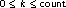. If MPI_GET_COUNT returns k, then the number of basic elements received (and the value returned by MPI_GET_ELEMENTS or MPI_GET_ELEMENTS_X) is n · k. If the number of basic elements received is not a multiple of n, that is, if the receive operation has not received an integral number of datatype ``copies,'' then MPI_GET_COUNT sets the value of count to MPI_UNDEFINED.

Example Usage of MPI_GET_COUNT and MPI_GET_ELEMENTS.

```...
CALL MPI_TYPE_CONTIGUOUS(2, MPI_REAL, Type2, ierr)
CALL MPI_TYPE_COMMIT(Type2, ierr)
...
CALL MPI_COMM_RANK(comm, rank, ierr)
IF (rank.EQ.0) THEN
CALL MPI_SEND(a, 2, MPI_REAL, 1, 0, comm, ierr)
CALL MPI_SEND(a, 3, MPI_REAL, 1, 0, comm, ierr)
ELSE IF (rank.EQ.1) THEN
CALL MPI_RECV(a, 2, Type2, 0, 0, comm, stat, ierr)
CALL MPI_GET_COUNT(stat, Type2, i, ierr)     ! returns i=1
CALL MPI_GET_ELEMENTS(stat, Type2, i, ierr)  ! returns i=2
CALL MPI_RECV(a, 2, Type2, 0, 0, comm, stat, ierr)
CALL MPI_GET_COUNT(stat, Type2, i, ierr)     ! returns i=MPI_UNDEFINED
CALL MPI_GET_ELEMENTS(stat, Type2, i, ierr)  ! returns i=3
END IF
```

The functions MPI_GET_ELEMENTS and MPI_GET_ELEMENTS_X can also be used after a probe to find the number of elements in the probed message. Note that the MPI_GET_COUNT, MPI_GET_ELEMENTS, and MPI_GET_ELEMENTS_X return the same values when they are used with basic datatypes as long as the limits of their respective count arguments are not exceeded.

Rationale.

The extension given to the definition of MPI_GET_COUNT seems natural: one would expect this function to return the value of the count argument, when the receive buffer is filled. Sometimes datatype represents a basic unit of data one wants to transfer, for example, a record in an array of records (structures). One should be able to find out how many components were received without bothering to divide by the number of elements in each component. However, on other occasions, datatype is used to define a complex layout of data in the receiver memory, and does not represent a basic unit of data for transfers. In such cases, one needs to use the function MPI_GET_ELEMENTS or MPI_GET_ELEMENTS_X. ( End of rationale.)